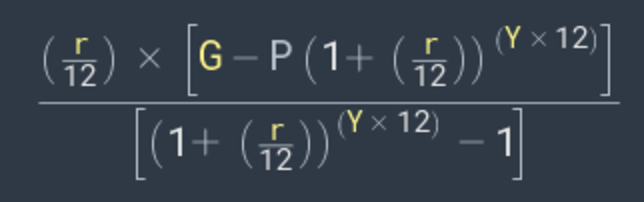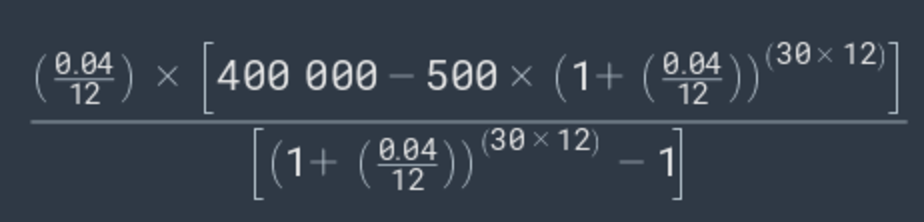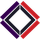## Savings Goal Calculator

This calculator tells how much money you need to save per day, month, or year to hit your savings goal. Factors in your current savings, an expected rate of return, and the number of years to save for.Answers to What You Seek

This calculator assumes that interest compounds monthly and that the interest rate is fixed.

Field summary:

• Savings Goal - how much money you want to have saved.
• Starting Amount - how much you currently have saved, can be negative if you are in debt.
• Growth Rate - this is the interest rate on your savings, also called the yield or the annual return on your savings.
• Years to Save - how many years you give yourself to meet your goal.

Some things to think about with savings:

• The higher the growth rate the higher the risk.  Remember there is no 'free lunch' on Wall Street, with the one exception - diversification. Having your assets spread across a multitude of asset classes as opposed to a few concentrated areas eliminates the possibility of getting rich quick, but makes your portfolio very efficient from a risk vs return standpoint.
• The more time you give yourself to save the better. Extra time gets the power of compound interest working in your favor.

The math:

In order to calculate what you would need to save per month to reach a savings goal, use the formula:r = annual interest rate, eg 7% = 0.07

G = savings goal

Y = number of years to save for

For example, if r = 4% (0.04), G = \$400,000, P = \$500, and Y = 30:Which equals \$537.94, just as the calculator shows!

DISCLAIMER: This calculator is provided for educational purposes and should not be considered financial or investment advice. We have checked the equations and code used and we think they are right. However, we offer you no guarantee of accuracy. If you find a bug please let us know so we can fix it for you!

To share this calculator by URL or embed it on your own website see the 'Share / Embed' button above next to the results bar after hitting Submit.Victor Feb 7 2021 00:39 UTC

Thank you for providing this calculator and also the formula. Can you kindly show me the formula used in calculating the amount to save daily?Feb 7 2021 01:15 UTC

@victor - this calculator compounds monthly. To arrive at the daily figure it takes the monthly figure (given by the equation in the image above), multiplies it by 12 (months / year), then divides by 365 (days / year) to get (dollars / day).

E.g. if the monthly is \$100, then the daily is (\$100 * 12) / 365 = 3.2876... (rounded to the nearest penny) = \$3.29.